# Transform Onto Curve

Using VEX to place packed primitive on curve and aligning primitive with curve normal.

There’s certainly other approaches – especially if you want to distribute lots of objects on a curve. I created this solution as a small part of a larger system where I want the user to be able to place data markers on a curve – and I like how the approach highlights using vectors to create a matrix in order to transform geometry.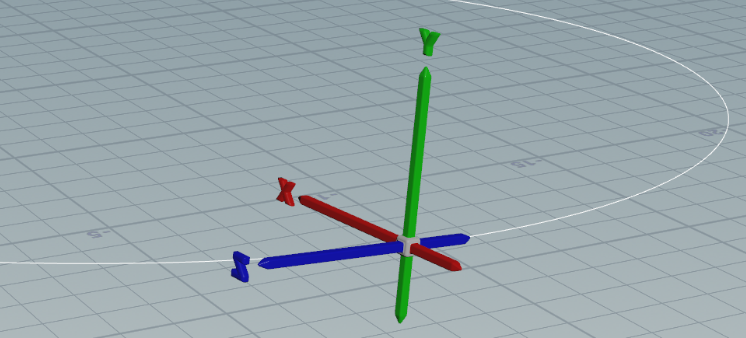### Curve Normal, Binormal, and Tangent

In this example we use a PolyFrame to add a tangent.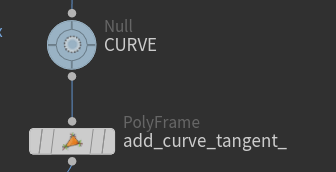The PolyFrame has these settings: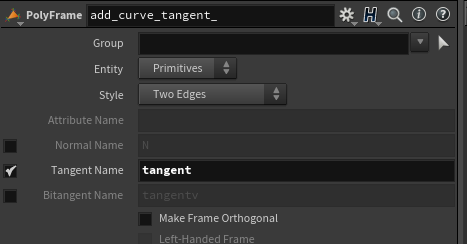Next we do a PointWrangle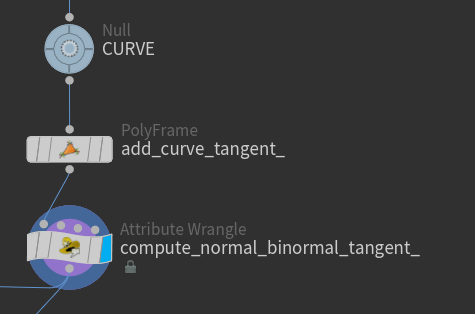Below VEX inverts the tangent so that it points “forward”; that is, from a lower indexed point towards a higher indexed point.

We also generate a binormal. This is orthogonal to the tangent in the horizontal plane.

A normal is generated from the tangent and binormal. This will be an “upward” vector that tilts with the vertical slope of the curve.

``````vector tangent = v@tangent;

tangent = normalize(tangent * -1);
vector binormal = cross(tangent, {0,1,0});
vector normal = cross(binormal, tangent);

v@tangent = tangent;
v@binormal = binormal;
v@normal = normal;``````

At this location of the graph each point has these attributes:

• P
• tangent (forward vector)
• binormal
• normal (upward vector)

### Transform Primitive

Now we bring in our packed primitive as input to an AttributeWrangle (named “transform_primitive_”) set to run over Detail. The curve is routed through input.

We merge the result of the AttributeWrangle and the curve so we can get a better view of what’s happening.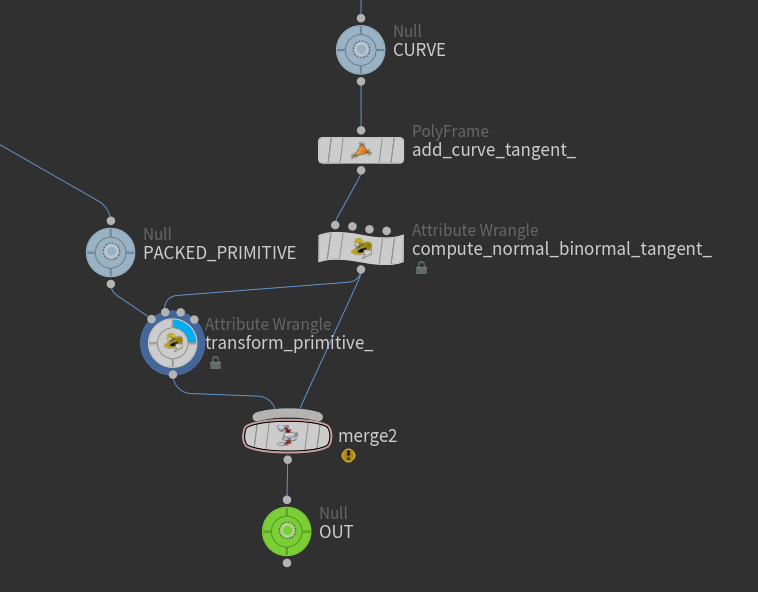``````/*
Parameter allowing user to select a parametric value between 0 and 1.
The parametric location value represents a location on the curve
expressed as a fraction of its length. A value of 0 represents the start
of the curve while 1 is the end of the curve.
*/
float location = ch("location");

// Using the location we compute the closest point on the curve.
int num_points = npoints(1);
float u_value = lerp(0, num_points - 1, location);
int point_num = int(rint(u_value));

/*
Now we collect information about that curve location.
If we want to place the packed primitive between points
along the the curve we could use primuv(1, "P", 0, uvw)
*/
vector position = point(1, "P", point_num);

vector tangent = point(1, "tangent", point_num);
vector binormal = point(1, "binormal", point_num);
vector normal = point(1, "normal", point_num);

// Create a matrix to transform with (rotation and scale)
matrix3 rotation_matrix = set(binormal, normal, tangent);
setprimintrinsic(0, "transform", 0, rotation_matrix);

// Translate the single point of the packed primitive
setpointattrib(0, "P", 0, position, "set");

// Geometry spreadsheet feedback for which point was used
i@point_num = point_num;``````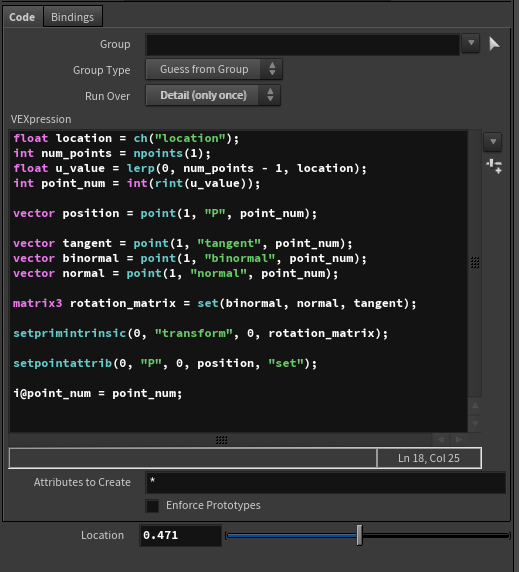Now you can visualize the OUT node and change the above Location parameter and see how the packed primitive is placed on and aligned with the curve.